# Comic Book Characters

Sara Stoudt true
05-29-2018

Data Source: FiveThirtyEight package

Article: FiveThirtyEight.com

``````require(dplyr)
require(ggplot2)
require(tidyr)

setwd("~/Desktop/tidytuesday/data/2018/2018-05-29/")
``````

#### Names: Boy v. Man, Girl v. Woman

``````cb\$isBoy <- unlist(lapply(cb\$name, function(x) {
grepl("boy\\>", x, ignore.case = T)
})) ## nothing after boy

cb\$isGirl <- unlist(lapply(cb\$name, function(x) {
grepl("girl", x, ignore.case = T)
})) ##

cb\$isMan <- unlist(lapply(cb\$name, function(x) {
grepl("man\\>", x, ignore.case = T)
})) ## nothing after man

cb\$isWoman <- unlist(lapply(cb\$name, function(x) {
grepl("woman", x, ignore.case = T)
})) ##

cb\$isMan[which(cb\$isMan == 1 & cb\$isWoman == 1)] <- 0 ## don't want to double count woman

byYear <- cb %>%
group_by(year) %>%
summarise(isGirl = sum(isGirl), count = n(), isWoman = sum(isWoman), isBoy = sum(isBoy), isMan = sum(isMan)) %>%
mutate(percentG = isGirl / count, percentW = isWoman / count)
``````

Tangent: Just for the record: characters identified as another’s girlfriend exist, but no boyfriends.

``````gf <- cb[which(unlist(lapply(cb\$name, function(x) {
grepl("girlfriend", x, ignore.case = T)
})) == T), ]
gf\$name
``````
`````` Ruby (Thug's girlfriend) (Earth-616)
 Annie (Noh-Varr's Girlfriend) (Earth-616)
 Karen (Hijack's girlfriend) (Earth-616)
23272 Levels: 'Spinner (Earth-616) ...``````
``````bf <- cb[which(unlist(lapply(cb\$name, function(x) {
grepl("boyfriend", x, ignore.case = T)
})) == T), ]
nrow(bf)
``````
`` 0``
``````sub <- byYear[, c("year", "isGirl", "isWoman")]
toPlotUpdated <- sub %>% gather(name, value, -year)

ggplot(toPlotUpdated, aes(x = year, y = value, col = name)) +
geom_point() +
geom_segment(aes(x = year, y = 0, xend = year, yend = value, col = name)) +
ylab("number of characters introduced with \n particular name") +
ggtitle("Where my girls at?") +
geom_text(aes(x = 1940.1, y = 1.2, label = "Wonder\n Woman"))
``````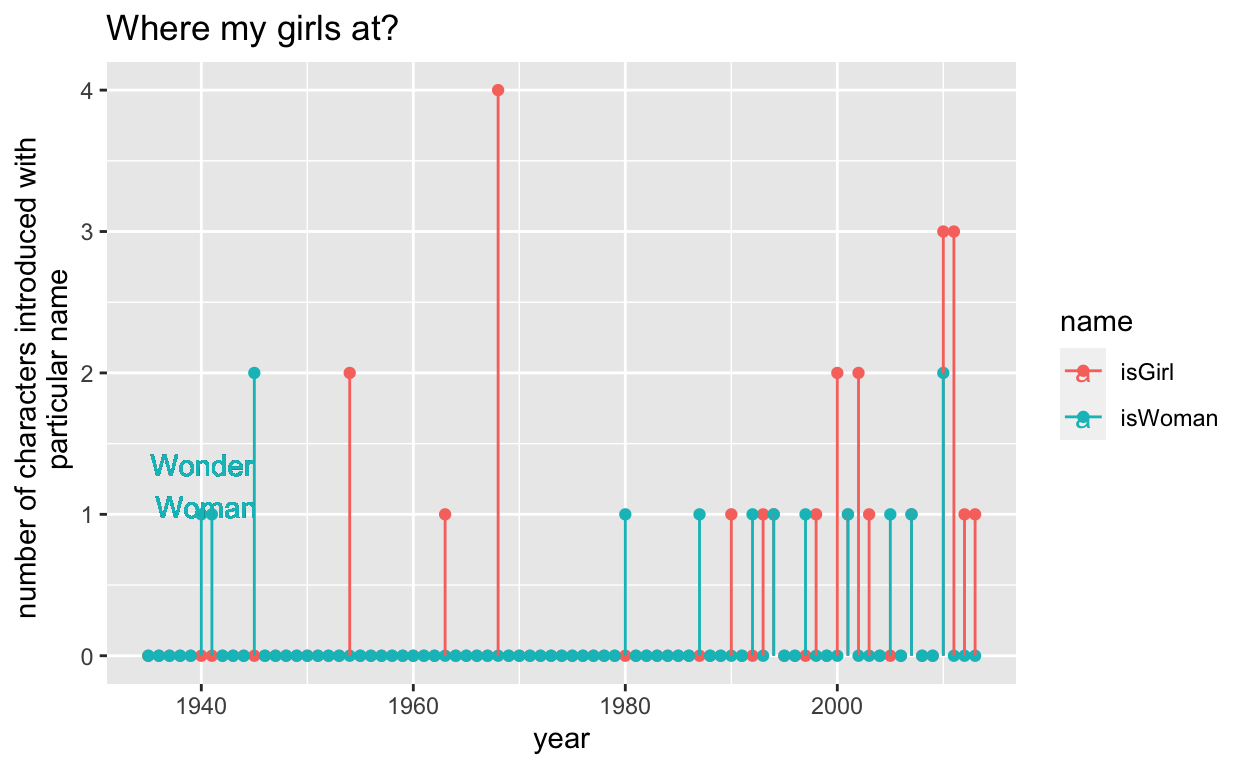``````sub <- byYear[, c("year", "isBoy", "isMan")]
toPlotUpdated <- sub %>% gather(name, value, -year)

ggplot(toPlotUpdated, aes(x = year, y = value, col = name)) +
geom_point() +
geom_segment(aes(x = year, y = 0, xend = year, yend = value, col = name)) +
ylab("number of characters introduced with \n particular name") +
ggtitle("It's a man's (super) world") +
geom_text(aes(x = 1986, y = 15, label = "Superman"))
``````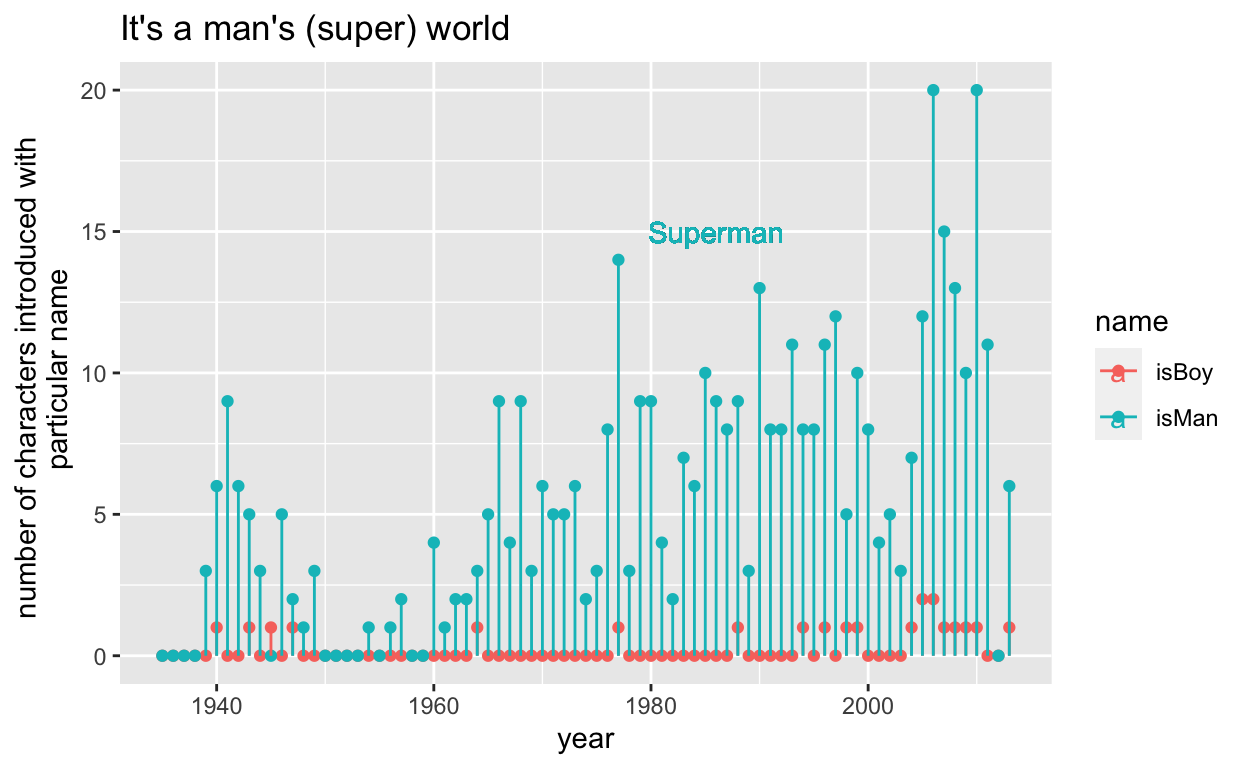Take-Away: There are way more “men” than “boys” but “girl” is pervasive even after the Wonder Woman precedent.

``````toPlot2 <- cb %>%
group_by(year) %>%
summarise(bad = length(which(align == "Bad Characters")), good = length(which(align == "Good Characters")))

toPlot2Update <- gather(toPlot2, align, count, -year)

ggplot(toPlot2Update, aes(x = year, y = count, col = align, group = align)) +
geom_point() +
geom_line() +
ylab("number of characters introduced")
``````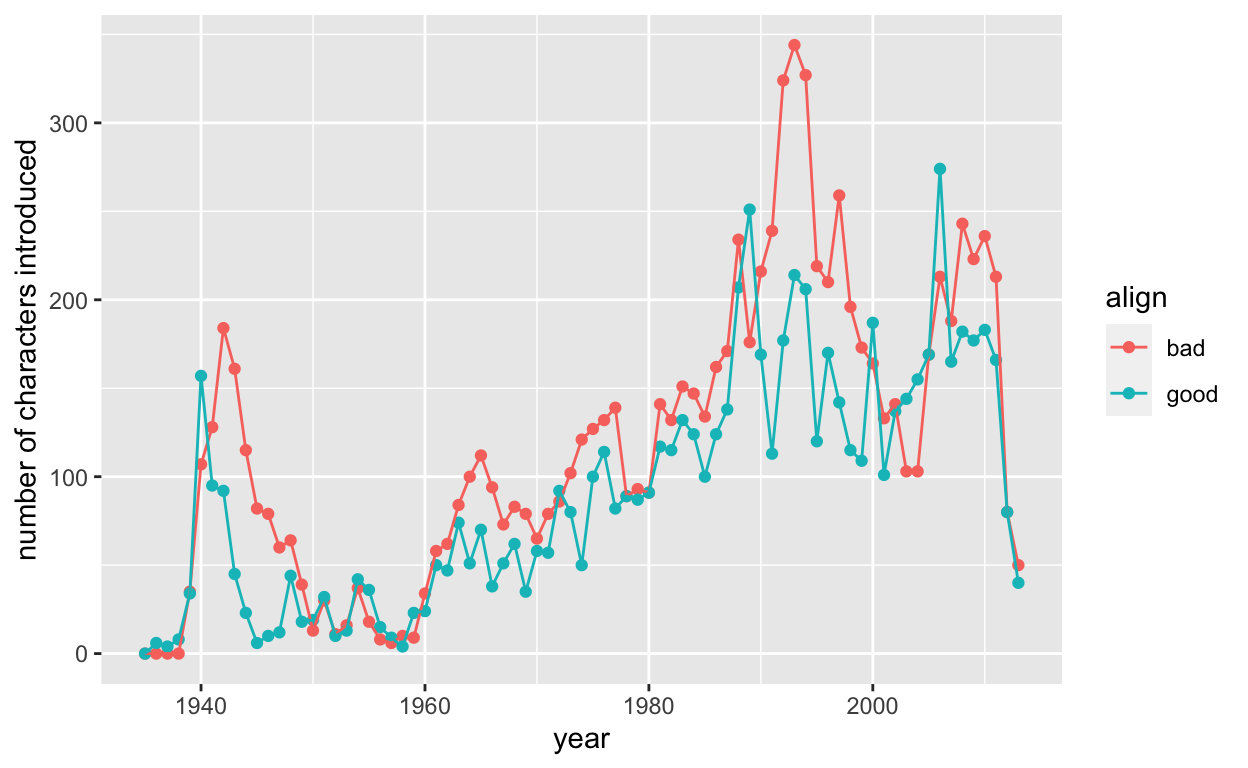``````ggplot(toPlot2, aes(x = year, y = bad / good)) +
geom_point() +
geom_hline(yintercept = 1) +
geom_text(aes(x = 1943, y = 10, label = "WWII")) +
ggtitle("A hero(ine) can punch above their weight")
``````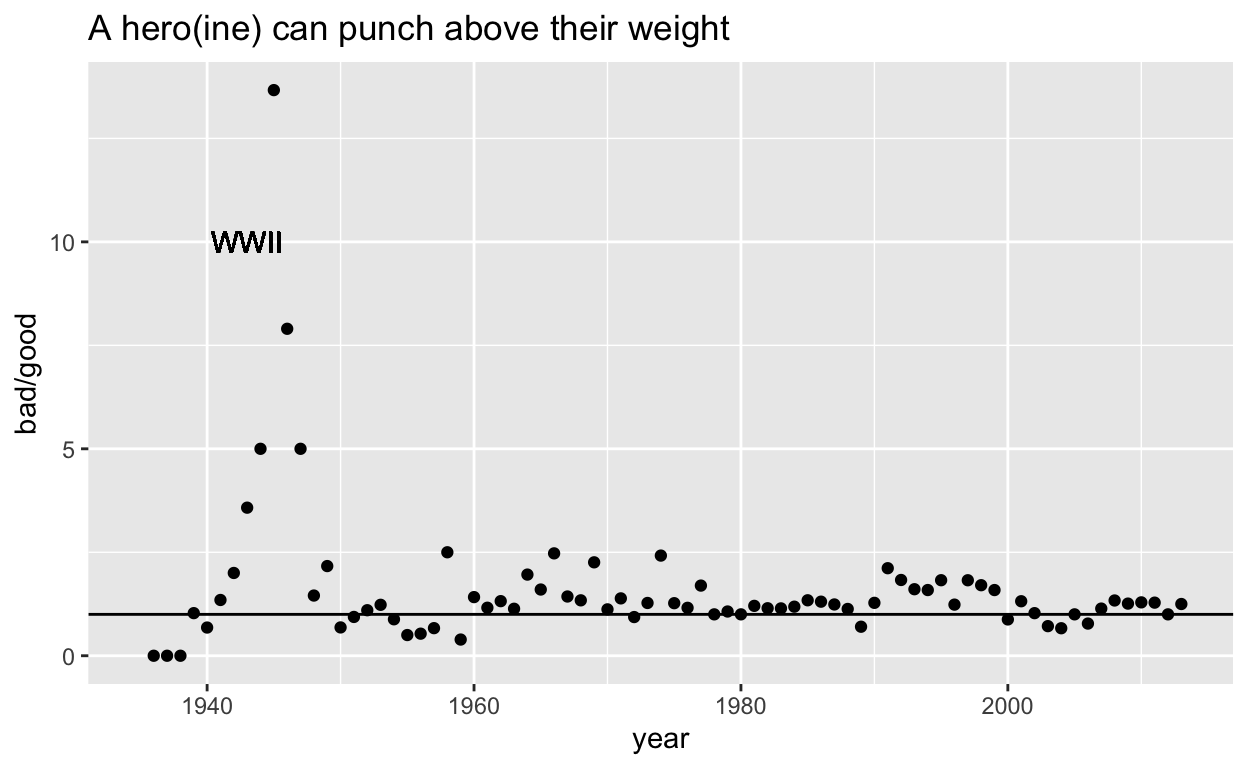Take-Away: More bad than good characters are introduced over time fairly consistently. Interestingly, there is a peak in bad characters during World War II. Overall, it looks like each hero can handle more than one villain.

#### Appearance Variability

``````toPlot <- cb %>%
group_by(year, sex) %>%
summarise(count = n(), uniqueEye = length(unique(eye)) / n(), uniqueHair = length(unique(hair)) / n()) %>%
filter(!is.na(sex)) %>%
filter(sex %in% c("Male Characters", "Female Characters"))

ggplot(toPlot, aes(x = year, y = uniqueEye, col = sex, group = sex)) +
geom_point() +
geom_line() +
ylab("number of unique eye color \n normalized by number of introductions")
``````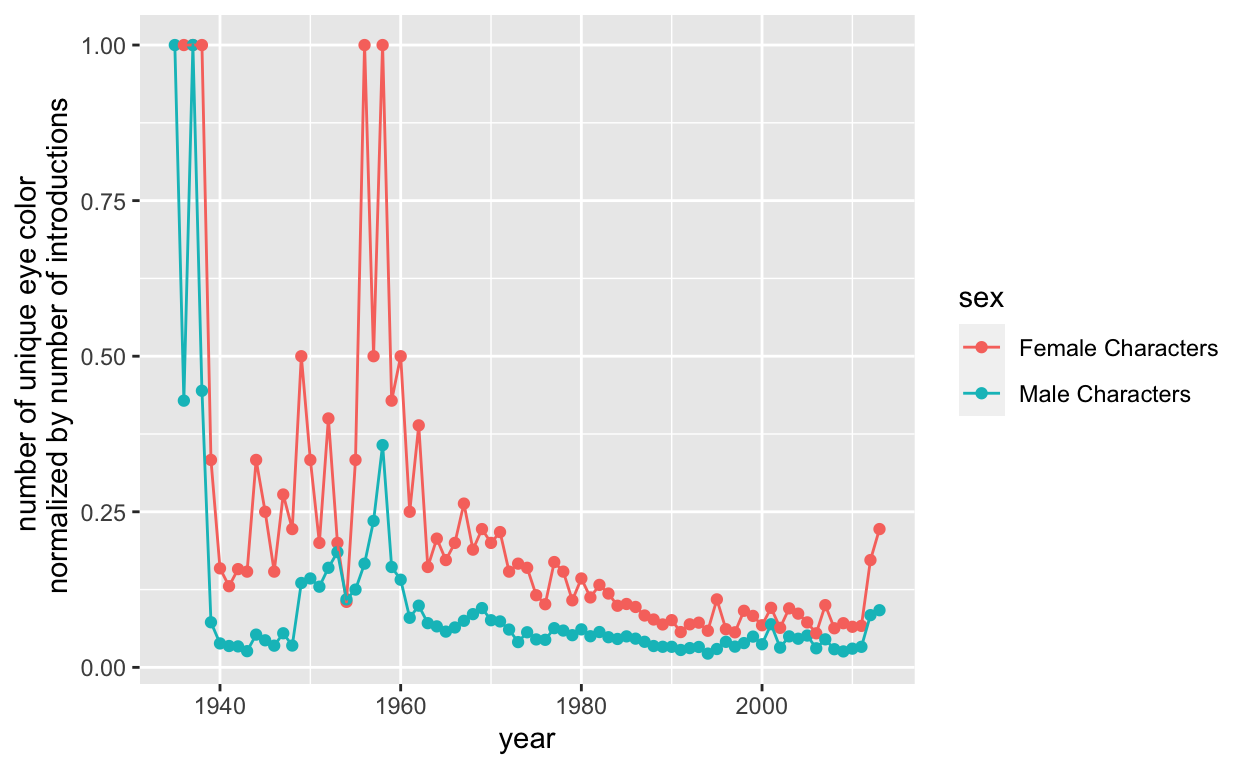``````ggplot(toPlot, aes(x = year, y = uniqueHair, col = sex, group = sex)) +
geom_point() +
geom_line() +
ylab("number of unique hair color \n normalized by number of introductions")
``````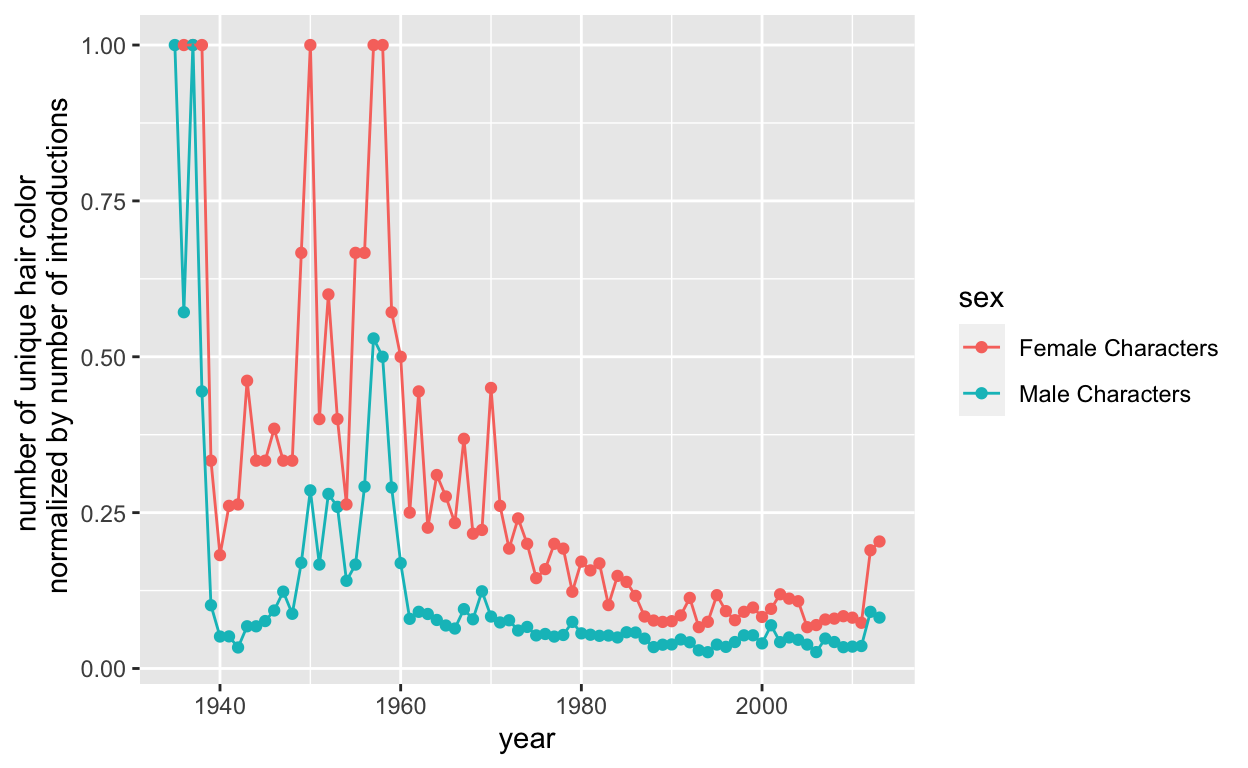``````ggplot(toPlot, aes(x = year, y = count, col = sex, group = sex)) +
geom_point() +
geom_line() +
ylab("number of introductions")
``````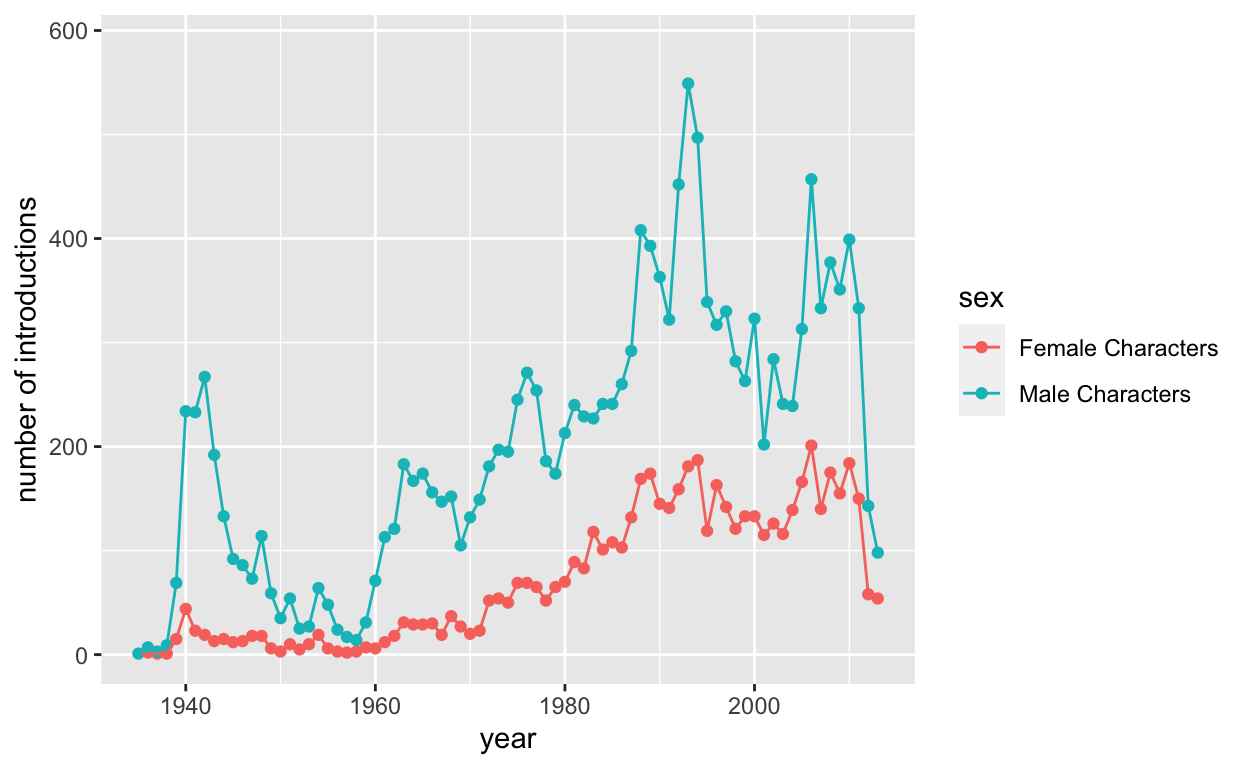Take-Away: There is (proportionally) more variability in hair and eye color for female characters. There is a decline in variability in hair and eye color over time, but at least part of this is due to the rise in new characters (and a limit on the number of hair/eye colors).

#### Identity by Sex

``````bySex <- cb %>%
group_by(sex) %>%
summarise(count = n())

bySexID <- cb %>%
group_by(sex, id) %>%
summarise(count = n()) %>%
inner_join(bySex, by = c("sex" = "sex")) %>%
mutate(percent = count.x / count.y)

toPlot <- bySexID %>%
filter(id %in% c("Public Identity", "Secret Identity", "No Dual Identity")) %>%
filter(!is.na(sex))

toPlot2 <- as.data.frame(toPlot %>% arrange(sex))

ggplot(toPlot2, aes(id, y = percent, fill = sex)) +
geom_bar(stat = "identity", position = position_dodge2(preserve = "total")) +
theme(axis.text.x = element_text(angle = 45, hjust = 1))
``````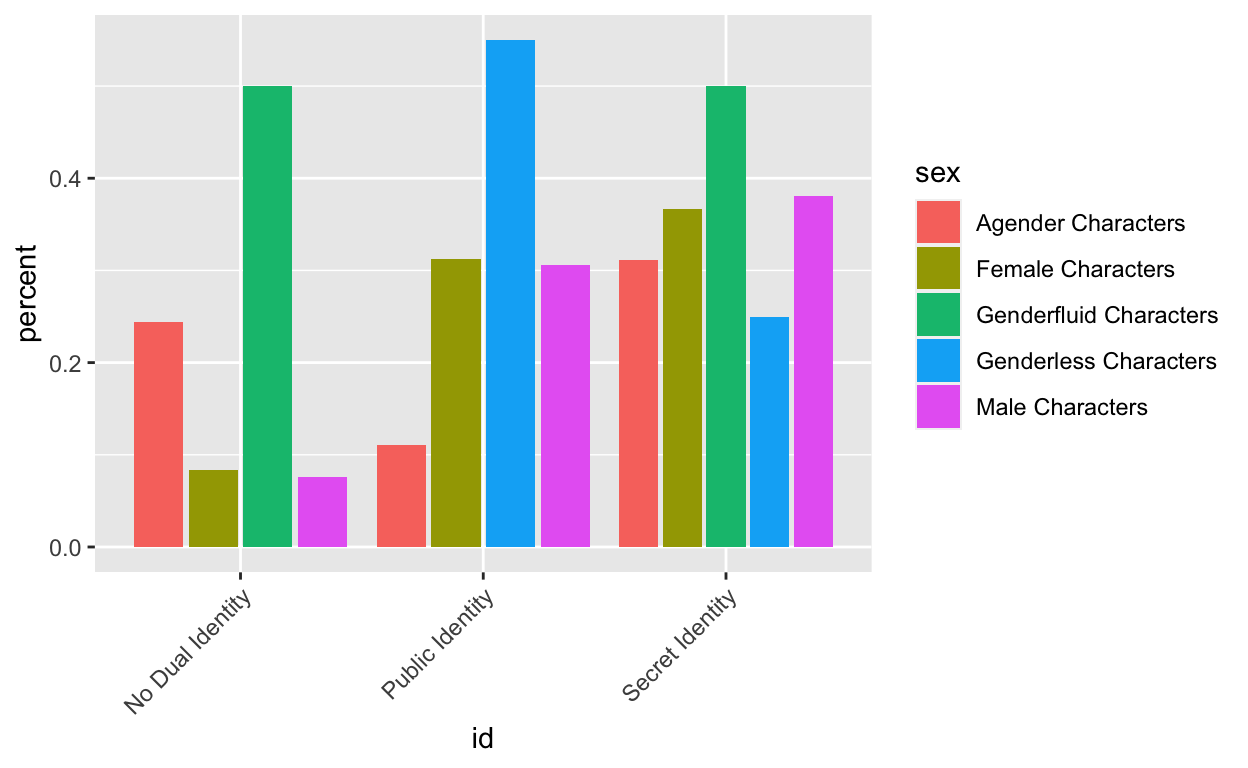Take-Away: There doesn’t seem to be any real difference between female and men in terms of identity. I am reluctant to make any claims about the other categories because of their small sample size.